# Storm and roof

The roof on the building is a cone with a height of 3 meters and a radius equal to half the height of the roof. How many m2 of roof need to be repaired if 20% were damaged in a storm?

x =  3.1612 m2

### Step-by-step explanation: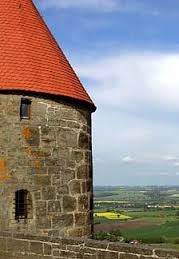Did you find an error or inaccuracy? Feel free to write us. Thank you!Tips to related online calculators
Pythagorean theorem is the base for the right triangle calculator.

#### You need to know the following knowledge to solve this word math problem:

We encourage you to watch this tutorial video on this math problem:

## Related math problems and questions:

• Cone roofHow many m2 of roofing is needed to cover a cone-shaped roof with a diameter of 10 m and a height of 4 m? Add an extra 4% to the overlays.
• Iglu - cone tentThe cone-shaped tent is 3 m high, the diameter of its base is 3.2 m. a) The tent is made of two layers of material. How many m2 of fabric is needed for production (including flooring) if 20% needs to be added to the minimum amount due to cutting waste? b)
• How manyHow many m2 of copper sheet is needed to replace the roof of a conical tower with a diameter of 13 meters and a height of 24 meters if we count 8% of the material for bending and waste?
• Church roof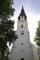The roof of the church tower has the shape of a regular tetrahedral pyramid with base edge length 5.4 meters and a height 5 m. It was found that needs to be corrected 27% covering of the roof area. What amount of material will be required?
• The roof of the church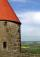The cone roof of the church has a diameter of 3 m and a height of 4 m. What is the size of the side edge of the church roof (s=?) and many sheets sheet will be needed to cover the church roof?
• Church roof 2The roof has the shape of a rotating cone shell with a base diameter of 6 m and a height of 2.5 m. How many monez (CZK) will cost the roof cover sheet if 1 m2 of metal sheet costs 152 CZK and if you need 15% extra for joints, overlays and waste?
• The diagram 2The diagram shows a cone with slant height 10.5cm. If the curved surface area of the cone is 115.5 cm2. Calculate correct to 3 significant figures: *Base Radius *Height *Volume of the cone
• Tiles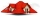The pupils of the building school have to calculate how many roof tiles they will need to cover the roof of the house in the shape of two rectangles measuring 10 x 7.2 m. One roof tile covers a rectangular area of 22 cmx32 cm. How many tiles will they nee
• Roof 7The roof has the shape of a regular quadrangular pyramid with a base edge of 12 m and a height of 4 m. How many percent is folds and waste if in construction was consumed 181.4m2 of plate?
• Pyramid roof2/4 of the area of ​​the roof-shaped regular tetrahedral pyramid with base edge 10 m and height of 4 m is already covered with roofing. How many square meters still need to be covered?
• Castle towerThe castle tower has a cone-shaped roof with a diameter of 10 meters and a height of 8 meters. Calculate how much m² of coverage is needed to cover it if we add one-third to the overlap.
• Rotating coneCalculate the volume and the surface area of a rotating cone of base radius r = 2.3 dm and a height h = 46 mm.
• TowerThe top of the tower is a regular hexagonal pyramid with base edge 8 meters long and a height 5 meters. How many m2 of the sheet is required to cover the top of the tower if we count 8% of the sheet waste?
• Lateral surface areaThe ratio of the area of the base of the rotary cone to its lateral surface area is 3: 5. Calculate the surface and volume of the cone, if its height v = 4 cm.
• House roofThe roof of the house has the shape of a regular quadrangular pyramid with a base edge 17 m. How many m2 is needed to cover roof if roof pitch is 57° and we calculate 11% of waste, connections and overlapping of area roof?
• The conical roof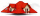The conical roof above the warehouse has a diameter of the lower part (base) d = 11.2 m and a height v = 3.3 m. How many rectangular steel plates with dimensions of 1.4 m and 0.9 m were needed for the production of this roof, if the seams and waste requir
• CanopyMr Peter has a metal roof cone shape with a height of 127 cm and radius 130 cm over well. He needs to paint the roof with anticorrosion. How many kg of color must he buy if the manufacturer specifies the consumption of 1 kg to 3.3 m2?